We are frequently asked, "I want to know the maximum value", "Is there a peak hold function?”,
"I want to know the maximum value of noise during a certain measurement time", etc.

Two parameters that represent the maximum value of sound in the sound level meter: One is the maximum value of time-weighted sound level. Another is peak sound level. Both values represent the maximum value of the sound generated within a certain time, however, the way of calculation and usage are different. In this column, I will explain these two value that are confused.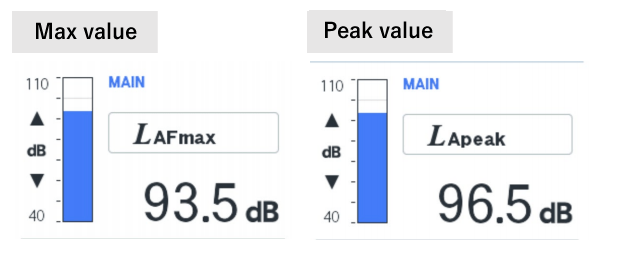The differences between the MAX value and the PEAK value are as follows:
→MAX value: the maximum time-weighted sound pressure level within a certain measurement time, relation to time-weighing

→Peak value: the maximum absolute value of the instantaneous sound pressure within a certain measurement time, no relation to time-weighting

The Sound level Lp is calculated by the following operation that is called “leveling”.
P0 is the root mean square value of the reference sound pressure（2×10-5 Pa） and the instantaneous sound pressure (sampled data).
(At the peak sound level, the maximum value of the absolute instantaneous sound pressure)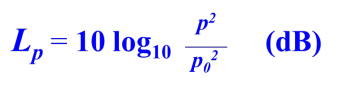In other words, the common logarithmic of the ratio to the reference sound pressure is taken and multiplied by 20.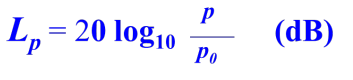#### What is Max Value?

When calculating the MAX value, the sound level meter processes the input sound signals as follow:

1. Apply frequency weighting (A, C, and Z weighting)
2. Apply time weighting (time constant: Fast, Slow) and root mean square
3. Calculate time-weighted sound level
4. Calculate the maximum value of sound level within a measurement time.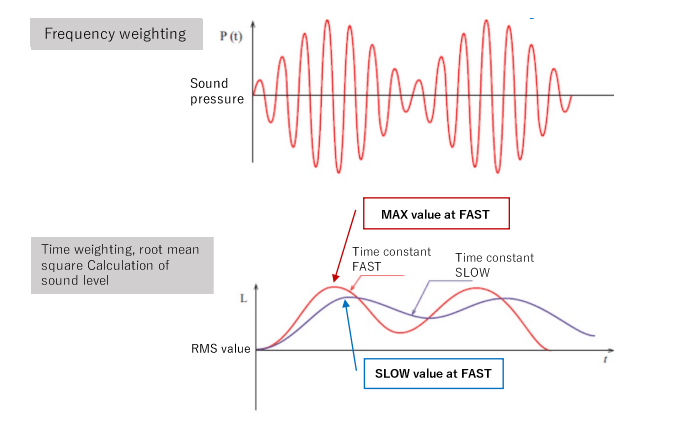Since the MAX value is the maximum value of the sound level (time-weighted sound level), its scale may change depending on the time constant when it is root mean squared.

I will explain further the effect of the difference in time constant on the MAX value. The time weighing is applied to the sound level when calculating the RMS value, thus it may not follow the rapid fluctuation of the sound.

The figure below shows sound level fluctuations when 1 kHz tone burst signals (duration: 200 ms) are input into Fast and Slow time constants. In contrast to the FAST (time constant: 125 ms), the SLOW (time constant: 1s) takes a longer time to rise the level value, thus it has no signals in the middle of rising, and difference in the measured value with the Fast.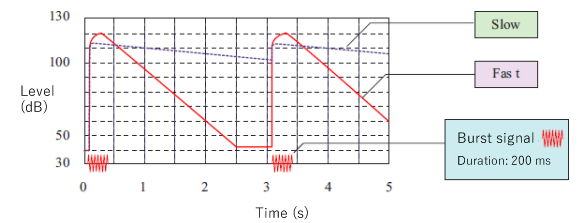#### What is Peak Value?

When calculating the Peak value, the sound level meter processes the input sound signals as follow:

1. Apply frequency weighting (A, C, and Z weighting)
2. Calculate the absolute value of instantaneous sound pressure
3. Calculate the maximum value of the absolute instantaneous sound pressure within a measurement time.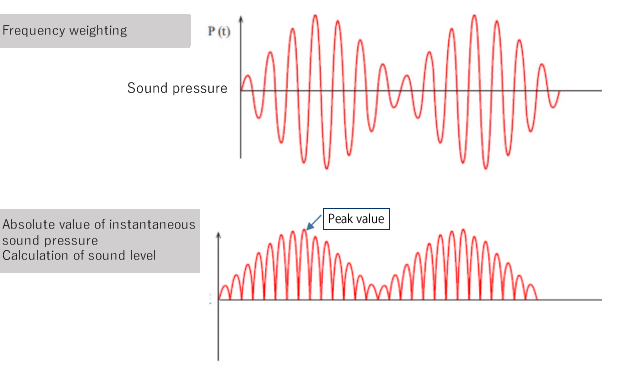The PEAK value is the level of the maximum instantaneous sound pressure value within a certain measurement time without calculating the RMS value, so it is not affected by the time weighting. Lpeak> Lmax, and there is a difference of about 3 dB for a sine wave signal.

#### MAX value and PEAK value

For instantaneous time, like impulsive sounds, the difference between the MAX and PEAK values is much greater such as 10 dB or more. The figure below shows the comparison between the waveform with leveled RMS value and the waveform with leveled absolute value of sound pressure for the signals with a duration of about 250 μs.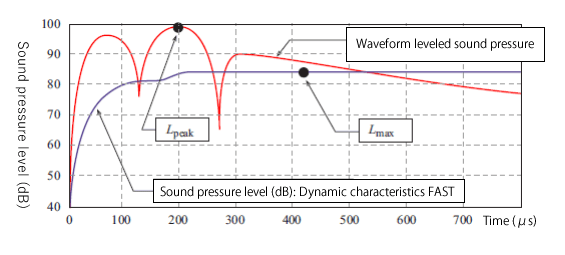Both the MAX and PEAK values represent the maximum values in a certain time, however, the usage of purpose is different.

The MAX value is used to measure intermittent noise in environmental noise and proximity exhaust noise. Since the PEAK value captures instantaneous changes of sound pressure well, it is used to evaluate the impact resistance of noise and to measure the C-weighted peak sound level, LCpeak value for CE marking regulation.

If you want to measure the maximum noise level, you need to determine which of the PEAK and MAX values is the most appropriate measurement method, and then select the correct one. Note that you cannot obtain the PEAK value from the MAX value and vice versa.

Make sure to understand the differences of these values and to clarify what kind of data you need before you start measuring.

##### Supplement：Why is there a difference between the display value and the calculation result of the sound level meter?

Since the display of sound level meter is updated every second, the value changes from “display A” to “display B”. While, the level data is calculated at sampling interval. Example of sampling interval: LA-1411/1441A is 31.25μs, LA-4441A is 20.83 μs, LA-7000 series is 15.625 μs. Since the Lmax and Lpeak values are obtained from the data calculated at this sampling interval, they may be much different from the value indicated by the instantaneous value.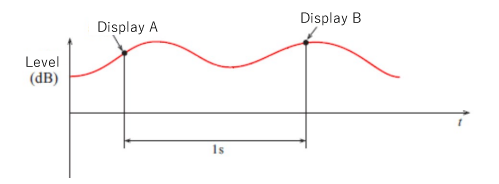(H·O)